# Olympiad Test: Pattern - 2

## 10 Questions MCQ Test Science Olympiad Class 8 | Olympiad Test: Pattern - 2

Description
Attempt Olympiad Test: Pattern - 2 | 10 questions in 20 minutes | Mock test for Class 8 preparation | Free important questions MCQ to study Science Olympiad Class 8 for Class 8 Exam | Download free PDF with solutions
QUESTION: 1

### 83 (40) 27 96 (81) 84 79 (?) 68

Solution: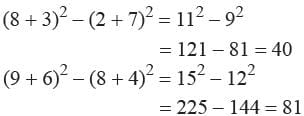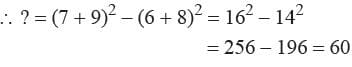QUESTION: 2

###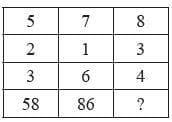Solution:

52 + 22 + 32 = 38
72 + 12 + 62 = 86
∴ ? = 82 + 32 + 42 = 89

QUESTION: 3

###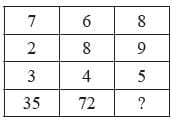Solution:

7 × (2 + 3) = 7 × 5 = 35
6 × (8 + 4) = 6 × 12 = 72
8 × (9 + 5) = 8 × 14 = 112

QUESTION: 4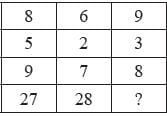Solution:

(8 – 5) × 9 = 3 × 9 = 27
(6 – 2) × 7 = 4 × 7 = 28
∴ ? = (9 – 3) × 8 = 6 × 8 = 48

QUESTION: 5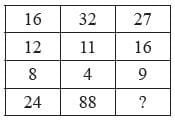Solution: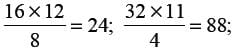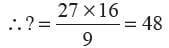QUESTION: 6

83 (52) 74 68 (63) 35 92 (?) 27

Solution:

(8 × 3) + (7× 4) = 24 + 28 = 52
(6 × 8) + (3 × 5) = 48 + 15 = 63
∴ ? = (9 × 2) + (2 × 7) = 18 + 14 = 32

QUESTION: 7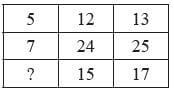Solution:

52 + 122 = 132
72 + 242 = 252
x2 + 152 = 172
⇒ x2 = 289 – 225 = 64 = 82
∴ x = 8

QUESTION: 8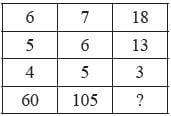Solution: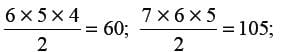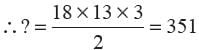QUESTION: 9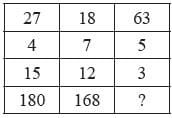Solution: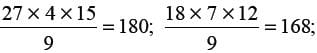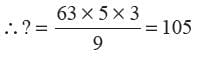QUESTION: 10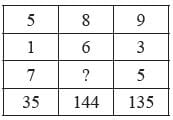Solution:

5 × 1 × 7 = 35
9 × 3 × 5 = 135
8 × 6 × x = 144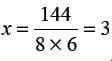Use Code STAYHOME200 and get INR 200 additional OFF Use Coupon Code